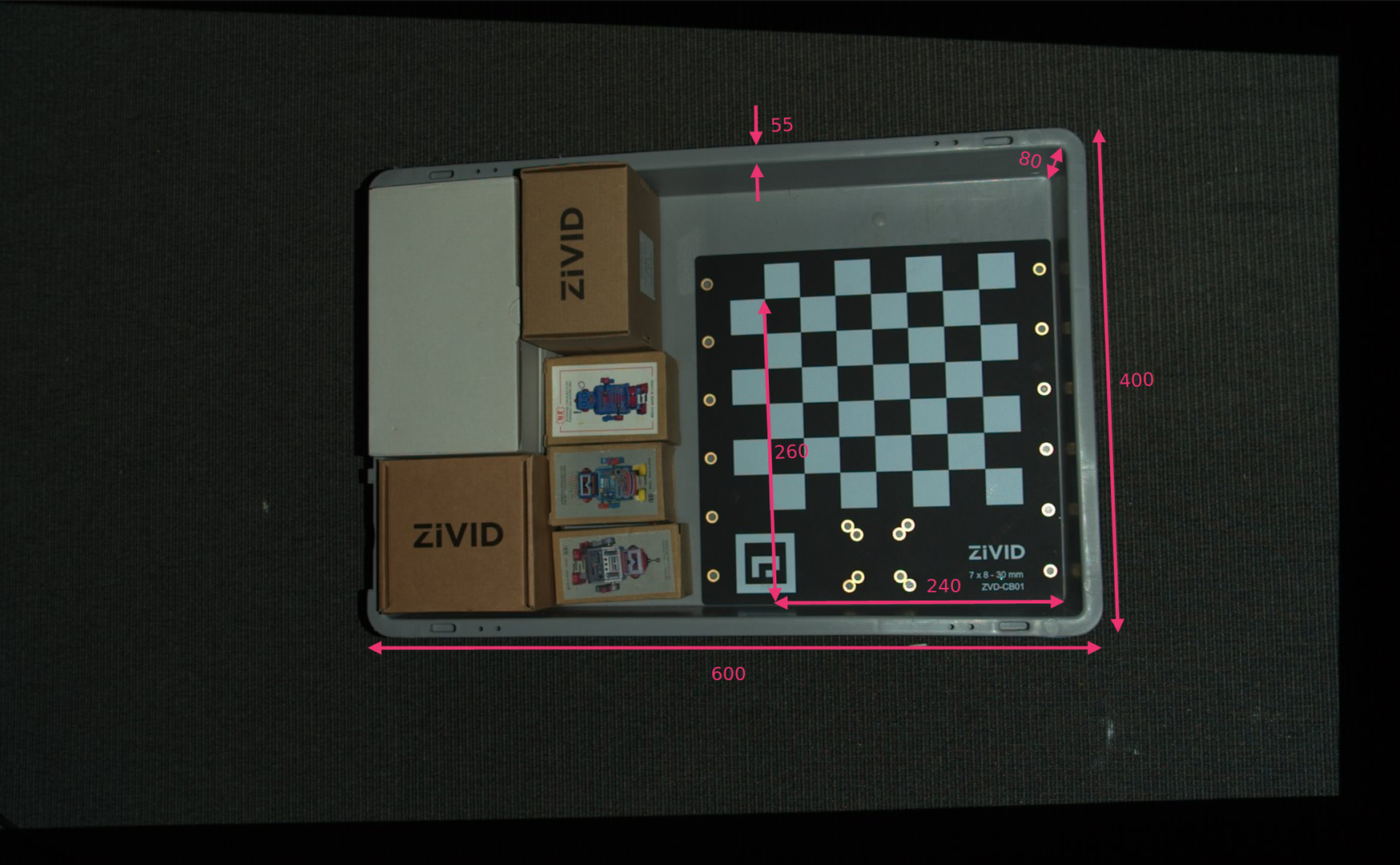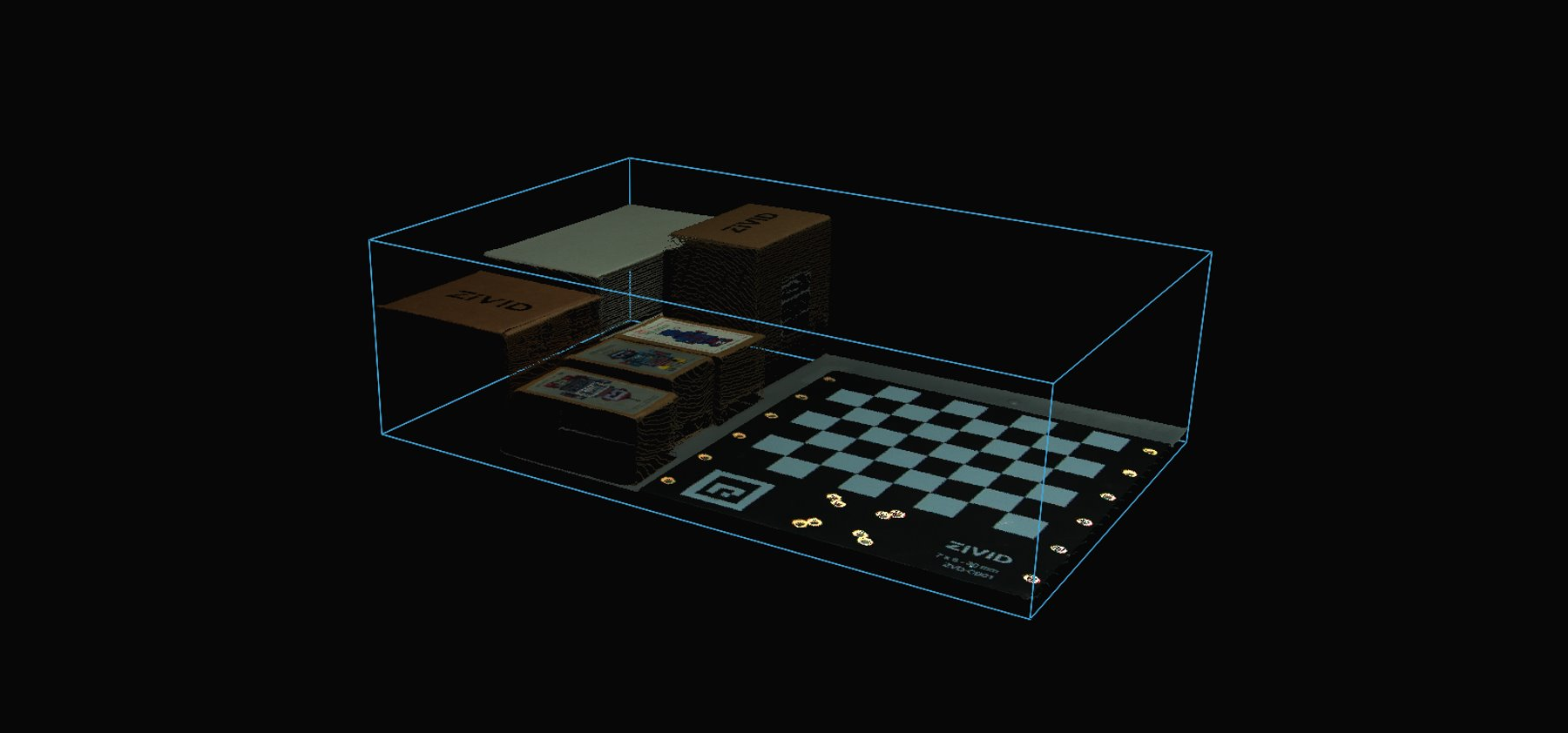# ROI box filter via Checkerboard

This tutorial demonstrates how to filter the point cloud transformed to the checkerboard coordinate system based on a ROI box given relative to the board. This results in filtering out all the points that are outside of the bin.

Tip

If you are using a Zivid One+ Small camera, the checkerboard might be too large for this application. For that case we have ROI box filter via ArUco marker tutorial.

This tutorial uses the point cloud of a checkerboard displayed in the image below.We can open the `original point cloud` in Zivid Studio and inspect it.

Note

The original point cloud is also in Sample Data.

To skip the part about transforming the point cloud, which is also covered in Transform via Checkerboard, go to Filtering based on ROI box.

Transforming the point cloud

First, we load a point cloud of a checkerboard.

```const auto fileData = std::string(ZIVID_SAMPLE_DATA_DIR) + "/BinWithCalibrationBoard.zdf";
std::cout << "Reading ZDF frame from file: " << fileData << std::endl;
const auto frame = Zivid::Frame(fileData);
auto pointCloud = frame.pointCloud();
```

Then we estimate the pose of the checkerboard and we invert it because we need the pose of the camera relative to the checkerboard.

```std::cout << "Detecting and estimating pose of the Zivid checkerboard in the camera frame" << std::endl;
const auto detectionResult = Zivid::Calibration::detectFeaturePoints(pointCloud);
const auto transformCameraToCheckerboard = detectionResult.pose().toMatrix();
std::cout << "Camera pose in checkerboard frame:" << std::endl;
const auto transformCheckerboardToCamera = transformCameraToCheckerboard.inverse();
std::cout << transformCheckerboardToCamera << std::endl;
```

We then transform the point cloud to the checkerboard coordinate system.

```std::cout << "Transforming point cloud from camera frame to Checkerboard frame" << std::endl;
pointCloud.transform(transformCheckerboardToCamera);
```

Filtering based on ROI box

Now we define the position of the bottom-left corner of the ROI box relative to the checkerboard frame, and the ROI box size.```std::cout << "Bottom-Left ROI Box corner:" << std::endl;
const float roiBoxBottomLeftCornerX = -80.F; // Positive is "East"
const float roiBoxBottomLeftCornerY = 280.F; // Positive is "South"
const float roiBoxBottomLeftCornerZ = 5.F;   // Positive is "Down"
std::cout << "X: " << roiBoxBottomLeftCornerX << std::endl
<< "Y: " << roiBoxBottomLeftCornerY << std::endl
<< "Z: " << roiBoxBottomLeftCornerZ << std::endl;
std::cout << "ROI Box size:" << std::endl;
const float roiBoxLength = 600.F;
const float roiBoxWidth = 400.F;
const float roiBoxHeight = 80.F;
std::cout << "Length: " << roiBoxLength << std::endl
<< "Width: " << roiBoxWidth << std::endl
<< "Height: " << roiBoxHeight << std::endl;
```

Now we filter the point cloud based on the size and position of the ROI box. The implementation is done using PCL library.

```std::cout << "Filtering the point cloud based on ROI Box" << std::endl;
const auto roiPointCloudPCL = roiBoxPointCloud(
pointCloud,
roiBoxBottomLeftCornerX,
roiBoxBottomLeftCornerY,
roiBoxBottomLeftCornerZ,
roiBoxLength,
roiBoxWidth,
roiBoxHeight);
```

Finally, we visualize the transformed and filtered point cloud.

```std::cout << "Displaying transformed point cloud after ROI Box filtering" << std::endl;
visualizePointCloud(roiPointCloudPCL);
```Lastly, we display the depth map of the transformed and filtered point cloud.

```std::cout << "Displaying depth map of the transformed point cloud after ROI Box filtering" << std::endl;
visualizeDepthMap(*roiPointCloudPCL);
```